# seapy.components.beam.SubsystemBend¶

class seapy.components.beam.SubsystemBend(name, system, **properties)[source]

Subsystem for bending waves in a 1D system.

__init__(name, system, **properties)

Constructor.

Parameters: name (string) – Identifier component (SeaPy.components.Component) – Component

Methods

 __init__(name, system, **properties) Constructor. addExcitation(name, model, **properties) Add excitation to subsystem. disable([couplings]) Disable this subsystem. enable([couplings]) Enable this subsystem. info([attributes]) Return dataframe. plot(quantity[, yscale]) Plot quantity.

Attributes

 SORT str(object=’‘) -> str average_frequency_spacing Average frequency spacing for bending waves. classname Name of class of the object. component conductance Conductance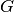. conductance_point_average Average point conductance of a structural component. damping_term The damping term is the ratio of the modal half-power bandwidth to the average modal frequency spacing. dlf Damping loss factor of subsystem. enabled Switch indicating whether the object is enabled. energy Total energy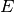in subsystem. flexural_rigidity Flexural rigidity or bending stiffness of a beam. frequency Frequency. impedance Impedance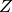impedance_point_force Impedance for bending waves in a thin beam excited far way from the edges. impedance_point_moment Moment impedance for bending waves excited in the center of the beam. included Indicates whether the object is included in the analysis. linked_couplings_from linked_couplings_to linked_excitations mobility Mobility Y modal_density Modal density. modal_energy Class capable of containing spectral values. modal_overlap_factor Modal overlap factor. name power_input Total input power due to excitations. resistance Resistance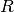, the real part of the impedance. resistance_point_average Average point resistance. soundspeed_group Group velocity for bending wave. soundspeed_phase Phase velocity for bending wave. tlf Total loss factor. velocity Vibrational velocity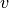. velocity_level Velocity level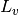. wavenumber Wave number.
average_frequency_spacing[source]

Average frequency spacing for bending waves. Valid when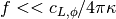.

Return type: numpy.ndarray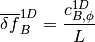See Lyon, eq. 8.1.10

flexural_rigidity[source]

Flexural rigidity or bending stiffness of a beam.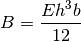with

• Young’s modulus• Beam height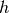• Beam width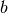:

See Craik, equation 3.3, page 48.

impedance_point_force[source]

Impedance for bending waves in a thin beam excited far way from the edges.

Return type: numpy.ndarray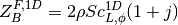See Lyon, table 10.1, second row.

impedance_point_moment[source]

Moment impedance for bending waves excited in the center of the beam.

Return type: numpy.ndarray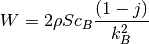See Lyon, table 10.2, second row.

soundspeed_group[source]

Group velocity for bending wave.

Return type: numpy.ndarray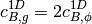soundspeed_phase[source]

Phase velocity for bending wave.

Return type: numpy.ndarray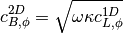See Lyon, above eq. 8.1.10

#### Previous topic

seapy.components.beam.SubsystemLong

#### Next topic

seapy.components.beam.SubsystemShear